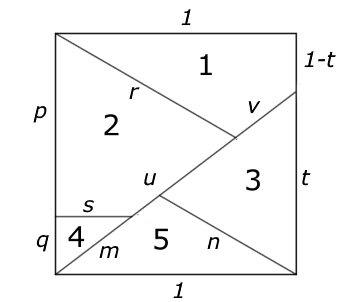#### You may also like### Degree Ceremony

What does Pythagoras' Theorem tell you about these angles: 90Â°, (45+x)Â° and (45-x)Â° in a triangle?### Logosquares

Ten squares form regular rings either with adjacent or opposite vertices touching. Calculate the inner and outer radii of the rings that surround the squares.### Ball Bearings

If a is the radius of the axle, b the radius of each ball-bearing, and c the radius of the hub, why does the number of ball bearings n determine the ratio c/a? Find a formula for c/a in terms of n.

# 30-60-90 Polypuzzle

##### Age 16 to 18Challenge Level
Here is a diagram showing five pieces of a puzzle that fit together to make a square.Can you re-arrange the pieces of the puzzle to form a rectangle by sliding the pieces without rotating them? Now can you re-arrange the pieces to form an equilateral triangle by flipping the pieces numbered $2$ and $5$ and moving them into new positions?

You can assume that pieces $1$ and $5$ each have a side of length one unit, that the pieces as shown form a perfect square of area one square unit and that they do fit together to form a perfect equilateral triangle of the same area.

Calculate the length $t$ of the edge of piece $3$ and then calculate the lengths of all the other edges giving answers correct to $3$ significant figures. You can use the interactivity below to explore how the pieces fit together.